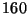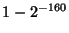Next: Questions about compare-by-hash Up: An Analysis of Compare-by-hash Previous: Traditional applications of hashing

# Compare-by-hash in detail

Compare-by-hash is a technique used when the payoff of discovering identical blocks is worth the computational cost of computing the hash of a block. In compare-by-hash, we assume hash collisions never occur, so we can treat the hash of a block as a unique id and compare only the hashes of blocks rather than the contents of blocks. For example, we can use compare-by-hash to reduce bandwidth usage. Before sending a block, the sender first transmits the hash of the block to the receiver. The receiver checks to see if it has a local block with the same hash value. If it does, it assumes that it is the same block as the sender's, without actually comparing the two input blocks. In the case of a 4096 byte block and a 160 bit hash value, this system can reduce network traffic from 4096 bytes to 20 bytes, or about a 99.5% savings in bandwidth.

This is an incredible savings! The cost, of course, is the risk of a hash collision. We can reduce that risk by choosing a collision-resistant hash. From a cryptographic point of view, collision resistance means that it is difficult to find two inputs that hash to the same output. By implication, the range of hash values must be large enough that a brute-force attack to find collisions is ``difficult.''2 Cryptographers have given us several algorithms that appear to have this property, although so far, only SHA-1 and RIPEMD-160 have stood up to careful analysis.

With a few assumptions, we can arrive at an estimate for the risk of a hash collision. We assume that the inputs to the hash function are random and uniformly distributed, and the output of the hash function is also random and uniformly distributed. Letbe the number of input blocks, and letbe the number of bits in the hash output. As a function of the number of input blocks,, the probability that we will encounter one or more collisions is. This is a difficult number to calculate whenis, but we can use the ``birthday paradox''3 to calculate how many inputs will give us a 50% chance of finding a collision. For a 160-bit output, we will need aboutorinputs to have a 50% chance of a collision. Put another way, we expect with about 48 nines () of certainty that any two randomly chosen inputs will not collide, whereas empirical measurements tell us we only have perhaps 8 or 9 nines of certainty that we will not encounter an undetected TCP error when we transmit the block. In the face of much larger sources of potential error, the error added by compare-by-hash appears to be negligible.

Now that we've described compare-by-hash in more detail, it should be clear how compare-by-hash and traditional hashing differ: No known previous uses of hashing skip the step of directly comparing the inputs for performance reasons. The only case in which we do skip that step is authentication, because we can't compare the inputs directly due to the lack of a secure channel. Compare-by-hash sets a new precedent and so does not yet enjoy the acceptance of established uses of hashing.Next: Questions about compare-by-hash Up: An Analysis of Compare-by-hash Previous: Traditional applications of hashing
2003-06-16